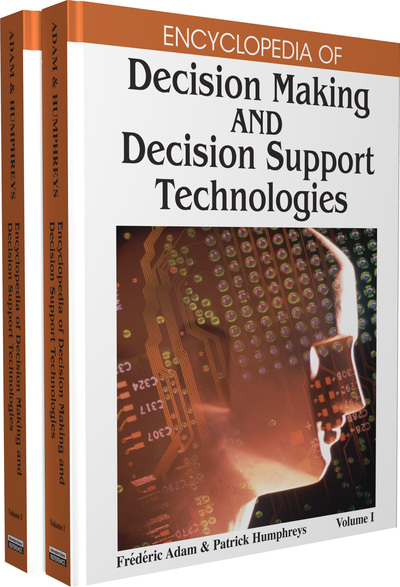# Dynamic System Simulation for Decision Support

Norman Pendegraft (University of Idaho, USA) and Mark Rounds (University of Idaho, USA)
DOI: 10.4018/978-1-59904-843-7.ch035

## Abstract

Simulation is a powerful methodology for decision support because it allows managers to experiment with models prior to implementing a policy or decision. There are several approaches to computer simulation: continuous event simulation, discrete event simulation, and Monte Carlo simulation. Continuous event simulation can be used to model dynamic system which cannot otherwise be easily modeled.

## Key Terms in this Chapter

Flow: An object in a dynamic systems simulation that represents changes in stocks.

Stock: An object in a dynamic systems simulation that represents some aspect of the state of system which may change over time.

Discrete Event Simulation: A simulation methodology in which events cause the state of the system to change at specific points in time.

Continuous Simulation: A simulation methodology in which uses the state of the system changes continuously in time.

Monte Carlo Simulation: A simulation in which random events are modeled using pseudo random number generators so that many replications of the random events may be evaluated statistically.

Simulation: Experimenting with a model (typically a computer model) of a system.

System Dynamics: A modeling approach developed by Forrester (1961) that models complex systems. An engineering discipline that deals with the mathematical analysis of dynamic systems.

Dynamic System: A system which has a state vector that changes over time.

## Complete Chapter List

Search this Book:
Reset